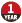# python 多叉树 import treelib / class 'generator' 的处理方法

2020-03-15 18:23 105 查看

### python 多叉树 import treelib / class 'generator' 的处理方法

1. 安装
```# windows
pip install treelib
# linux
sudo easy_install -U treelib```
```# 示例：
import treelib
from treelib import Tree, Node

class Nodex(object):
def __init__(self,x,y):
self.sit = [x,y]
tree = Tree()
tree.create_node('a', 1, data = Nodex(1,1))
tree.create_node('b', 2, parent = 1, data = Nodex(1,2))
tree.create_node('c', 3, parent = 1, data = Nodex(1,3))
tree.create_node('d', 4, parent = 2, data = Nodex(3,4))
tree.show()
tree.show(data_property = 'sit') #按data里的sit变量显示```
```#输出：
a
├── b
│   └── d
└── c

[1, 1]
├── [1, 2]
│   └── [3, 4]
└── [1, 3]```

treelib里有个函数rsearch(nid, filter=None)，它的作用是找到该节点到根的路径。

```rsearch(nid, filter=None)
# Traverse the tree branch along the branch from nid to its ancestors (until root).
# Parameters:filter – the function of one variable to act on the Node object.```

```a=tree.rsearch(4, filter=None)
print(a)
print(type(a))```
```#输出
<generator object Tree.rsearch at 0x0000000004F8D2C8>
<class 'generator'>```

generator保存的是算法，每次调用next()，就计算出下一个元素的值，直到计算到最后一个元素，没有更多的元素时，抛出StopIteration的错误。

```a=tree.rsearch(4, filter=None)
p=[]
while True:
try:
p.append(next(a))
except StopIteration as e:
break
print(p)```
```#输出
[4, 2, 1]```

• 点赞
• 收藏
• 分享
• 文章举报萝卜upupup 发布了7 篇原创文章 · 获赞 1 · 访问量 54 私信 关注